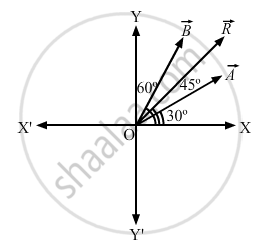Department of Pre-University Education, KarnatakaPUC Karnataka Science Class 11

# Let → a and → B Be the Two Vectors of Magnitude 10 Unit Each. If They Are Inclined to the X-axis at Angle 30° and 60° Respectively, Find the Resultant. - Physics

Let $\vec{A} \text { and } \vec{B}$ be the two vectors of magnitude 10 unit each. If they are inclined to the X-axis at angle 30° and 60° respectively, find the resultant.

#### Solution

Angle between $\vec{A} \text { and } \vec{B}$,θ = 60° − 30° = 30°

$\left| \vec{A} \right| = \left| \vec{B} \right| = 10 \text { units }$

The magnitude of the resultant vector is given by

$R = \sqrt{A^2 + A^2 + 2AA\cos\theta}$

$= \sqrt{{10}^2 + {10}^2 + 2 \times 10 \times 10 \times \cos 30^\circ}$

$= \sqrt{200 + 200 \cos 30^\circ}$

$= \sqrt{200 + 100}$

$= \sqrt{300} = 17 . 3 \text { units }$Let β be the angle between

$\vec{R} \text { and } \vec{A}$.

$\therefore \beta = \tan^{- 1} \left( \frac{A \sin 30^\circ}{A + A \cos 30^\circ} \right)$

$\Rightarrow \beta = \tan^{- 1} \left( \frac{10 \sin 30^\circ}{10 + 10 \cos 30^\circ} \right)$

$\Rightarrow \beta = \tan^{- 1} \left( \frac{1}{2 + \sqrt{3}} \right) = \tan^{- 1} \left( \frac{1}{3 . 372} \right)$

$\Rightarrow \beta = \tan^{- 1} \left( 0 . 26795 \right) = 15^\circ$

Angle made by the resultant vector with the X-axis = 15° + 30° = 45°
∴ The magnitude of the resultant vector is 17.3 and it makes angle of 45° with the X-axis.

Concept: What is Physics?
Is there an error in this question or solution?
Chapter 2: Physics and Mathematics - Exercise [Page 29]

#### APPEARS IN

HC Verma Class 11, Class 12 Concepts of Physics Vol. 1
Chapter 2 Physics and Mathematics
Exercise | Q 2 | Page 29
Share